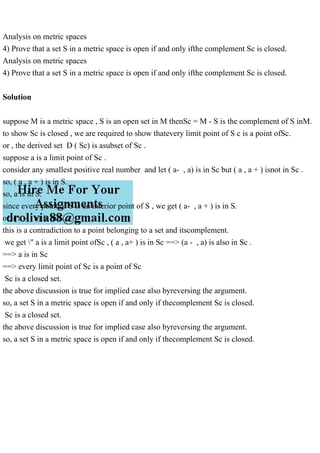PublicitéProchain SlideShareElements of Point Set Topology.pdf
Chargement dans ... 3
1 sur 1
Publicité

### Analysis on metric spaces4) Prove that a set S in a metric space.pdf

1. Analysis on metric spaces 4) Prove that a set S in a metric space is open if and only ifthe complement Sc is closed. Analysis on metric spaces 4) Prove that a set S in a metric space is open if and only ifthe complement Sc is closed. Solution suppose M is a metric space , S is an open set in M thenSc = M - S is the complement of S inM. to show Sc is closed , we are required to show thatevery limit point of S c is a point ofSc. or , the derived set D ( Sc) is asubset of Sc . suppose a is a limit point of Sc . consider any smallest positive real number and let ( a- , a) is in Sc but ( a , a + ) isnot in Sc . so, ( a , a + ) is in S. so, a is in S. since every point of S is an interior point of S , we get ( a- , a + ) is in S. or, ( a - , a) is in S. this is a contradiction to a point belonging to a set and itscomplement. we get " a is a limit point ofSc , ( a , a+ ) is in Sc ==> (a - , a) is also in Sc . ==> a is in Sc ==> every limit point of Sc is a point of Sc Sc is a closed set. the above discussion is true for implied case also byreversing the argument. so, a set S in a metric space is open if and only if thecomplement Sc is closed. Sc is a closed set. the above discussion is true for implied case also byreversing the argument. so, a set S in a metric space is open if and only if thecomplement Sc is closed.
Publicité